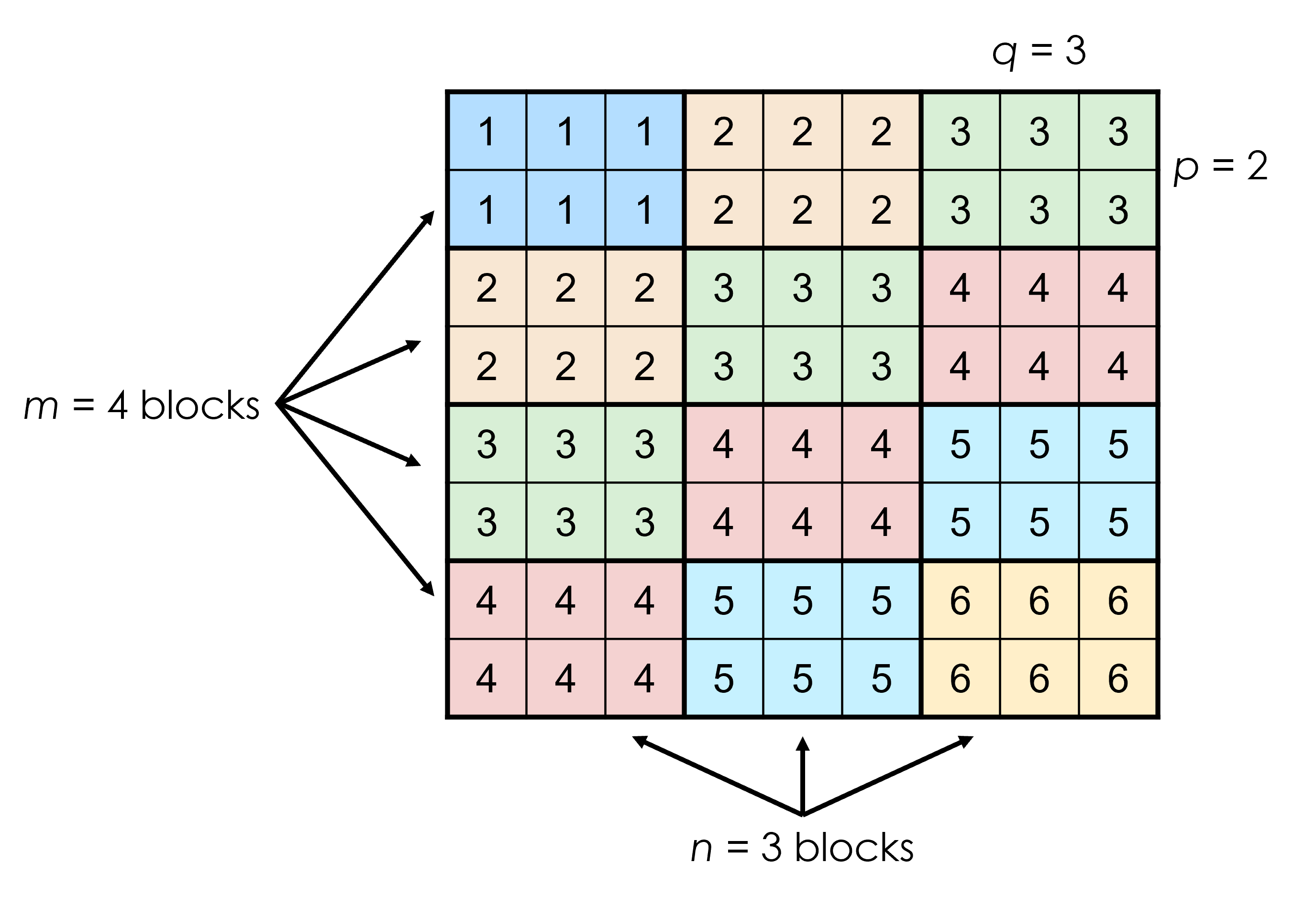# Problem 55255. Create block matrix of integers (j+k-1) - Part I

Given m, n, p, and q, create a matrix of m-by-n blocks (submatrices), each sized p-by-q. The elements of the (j,k)th block all have the same value: (j+k-1).
For example, if m = 4, n = 3, p = 2, and q = 3, the matrix is:You can assume m, n, p, and q are all positive integers. (They can have the value 1, however.)

### Solution Stats

69.39% Correct | 30.61% Incorrect
Last Solution submitted on Dec 03, 2023

### Community Treasure Hunt

Find the treasures in MATLAB Central and discover how the community can help you!

Start Hunting!